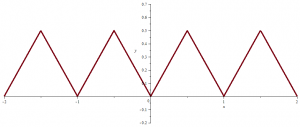# A differentiable function except at one point with a bounded derivative

We build here a continuous function of one real variable whose derivative exists except at $$0$$ and is bounded on $$\mathbb{R^*}$$.

We start with the even and piecewise linear function $$g$$ defined on $$[0,+\infty)$$ with following values:
$g(x)= \left\{ \begin{array}{ll} 0 & \mbox{if } x =0\\ 0 & \mbox{if } x \in \{\frac{k}{4^n};(k,n) \in \{1,2,4\} \times \mathbb{N^*}\}\\ 1 & \mbox{if } x \in \{\frac{3}{4^n};n \in \mathbb{N^*}\}\\ \end{array} \right.$
The picture below gives an idea of the graph of $$g$$ for positive values. Continue reading A differentiable function except at one point with a bounded derivative

# A continuous function which is not of bounded variation

## Introduction on total variation of functions

Recall that a function of bounded variation, also known as a BV-function, is a real-valued function whose total variation is bounded (finite).

Being more formal, the total variation of a real-valued function $$f$$, defined on an interval $$[a,b] \subset \mathbb{R}$$ is the quantity:
$V_a^b(f) = \sup\limits_{P \in \mathcal{P}} \sum_{i=0}^{n_P-1} \left\vert f(x_{i+1}) – f(x_i) \right\vert$ where the supremum is taken over the set $$\mathcal{P}$$ of all partitions of the interval considered. Continue reading A continuous function which is not of bounded variation

# A function that is everywhere continuous and nowhere differentiable

Let $$f_1(x) = |x|$$ for $$| x | \le \frac{1}{2}$$, and let $$f_1$$ be defined for other values of $$x$$ by periodic continuation with period $$1$$. $$f_1$$ graph looks like following picture:$$f_1$$ is continuous everywhere and differentiable on $$\mathbb{R} \setminus \mathbb{Z}$$. Continue reading A function that is everywhere continuous and nowhere differentiable

# An unbounded positive continuous function with finite integral

Consider the piecewise linear function $$f$$ defined on $$[0,+\infty)$$ taking following values for all $$n \in \mathbb{N^*}$$:
$f(x)= \left\{ \begin{array}{ll} 0 & \mbox{if } x=0\\ 0 & \mbox{if } x=n-\frac{1}{2n^3}\\ n & \mbox{if } x=n\\ 0 & \mbox{if } x=n+\frac{1}{2n^3}\\ \end{array} \right.$

The graph of $$f$$ can be visualized in the featured image of the post. Continue reading An unbounded positive continuous function with finite integral

# A continuous differential equation with no solution

Most of Cauchy existence theorems for a differential equation
\begin{equation}
\textbf{x}^\prime = \textbf{f}(t,\textbf{x})
\end{equation} where $$t$$ is a real variable and $$\textbf{x}$$ a vector on a real vectorial space $$E$$ are valid when $$E$$ is of finite dimension or a Banach space. This is however not true for the Peano existence theorem. Continue reading A continuous differential equation with no solution

# A nowhere continuous function

This is a strange function!

One example is the Dirichlet function $$1_{\mathbb{Q}}$$.
$$1_{\mathbb{Q}}(x)=1$$ if $$x \in \mathbb{Q}$$ and
$$1_{\mathbb{Q}}(x)=0$$ if $$x \in \mathbb{R} \setminus \mathbb{Q}$$.

$$1_{\mathbb{Q}}$$ is everywhere discontinuous because $$\mathbb{Q}$$ is everywhere dense in $$\mathbb{R}$$.

The function $$x \mapsto x \cdot 1_{\mathbb{Q}}(x)$$ is continuous in $$0$$ and discontinuous elsewhere.